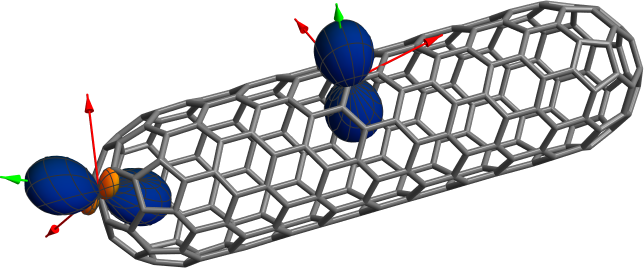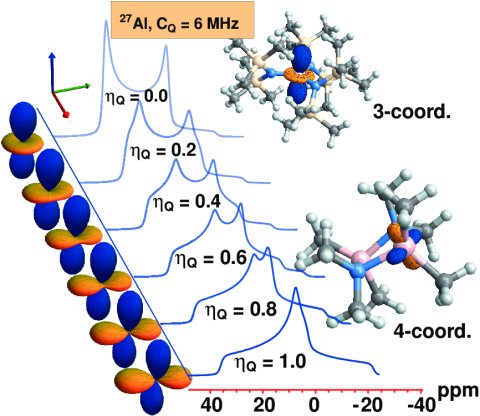#### Graphical representations of rank-2 tensors

Before we discuss the details, here is an example along with a brief description. The language used in the description is explained in detail further below. The figure was created with this Mathematica notebook (60 kBytes). For further details see my Downloads page.Graphical representations of selected NMR carbon shielding tensors of a finite (9,0) single-walled carbon nanotube. The blue (+) and orange () surfaces indicate the signs and relative magnitudes of the principal components of the NMR shielding tensors as well as the orientations of the principal axis systems (PASs) relative to the molecular coordinate frame. The arrows additionally show the PASs and are color coded (green for positive principal components and red for negative ones). A sphere would indicate an isotropic tensor. The nanotube shielding tensors are strongly anisotropic. Data to prepare the figure were taken from publication .

Many optical and spectroscopic properties of molecules that we study in our research, on a day-to-day basis or occasionally, are rank-2 tensors. Examples are NMR shielding, NMR spin-spin coupling, electric field gradients at nuclei (giving rise to the quadrupolar interaction in NMR and other types of spectroscopy), polarizability, optical rotation, or the magnetic susceptibility. If you know what a rank-2 tensor is please just scroll down to the detailed description of the functions that are plotted in the figure shown above. Otherwise, here is a short explanation:

What is a tensor, and what is a rank-2 tensor? A rank-0 tensor is a scalar (a single number). A rank-1 tensor is a vector. For a physical quantity defined in a 3D Cartesian coordinate system such a vector has three components:

 v =A rank-2 tensor can be written as a matrix. For example, for a quantity defined in a 3D Cartesian coordinate system a rank-2 tensor can be written as (we use boldface notation for vectors and boldface with a bar for rank-2 tensors here):

 M =In a nutshell, a tensor of rank greater than zero is a physical quantity that is represented by more than a single number, the tensor components. These components transform among each other under a transformation of the coordinate system such that physical laws written in terms of the tensors remain the same in different coordinate systems.

For example, the interaction energy (a scalar = rank-0 tensor) between a magnetic field B of magnitude B (i.e. B is the length of the vector B) and a magnetic dipole moment m with magnitude m is given by the dot-product of the two vectors:

 E = −m⋅B = −(mxBx + myBy + mzBz) = −mB cos[∠(m,B)]

The equations assume a Cartesian coordinate system with unit vectors ex,ey,ez of length 1. The point is that if we rotate the coordinate system used to describe the vectors B and m (passive rotation), or if the rotate the two vectors simultaneously in the exact same way (active rotation), the energy E must remain the same as we have neither changed the angle (m,B) between the two vectors nor their lengths. This puts certain constraints on how the components of the vectors must transform under rotations of the coordinate system or when they are rotated. Likewise, the components of a rank-2 or higher tensor have certain transformation rules upon rotations.

The previous equation for E is a good starting point to introduce a rank-2 tensor. If the magnetic dipole moment is that of an atomic nucleus’ spin, the energy E is quantized and we can observe transitions between ‘parallel’ and ‘anti-parallel’ relative orientations of B and m by spectroscopy. The magnetic field B is then the field created by the powerful magnet of an NMR spectrometer. But there is something else going on: there are electrons around a magnetic nucleus in a molecule, and there are other atoms around, and there are other molecules around those. Therefore the magnetic field experienced by the nuclear spin magnetic moment is not the field generated by the spectrometer magnet but a local field. The local field may differ in magnitude and orientation from the external spectrometer field, and it may depend on the relative orientations of the external field and the molecule. Usually, the magnitude of the local field is reduced relative to the external field, and the effect is therefore referred to as NMR shielding. But how to describe it theoretically?

Let’s denote the external spectrometer field by Bext and the local field by Bloc. Considering that the local field will usually be smaller in magnitude (hence, one needs a subtraction of some sort), we can write

 Bloc = (1 −σ)Bext = Bext −σBext

Here, σ is the nuclear magnetic shielding tensor. If σ were a scalar, then σBext would always be parallel to Bext, and therefore Bloc would also always be parallel to Bext. This is not general enough to describe experimental observations. Instead, NMR shielding is a rank-2 tensor:

 σ =The interaction energy between the nuclear magnetic moment and the magnetic field is

 E = m⋅Bloc = m⋅ (1 −σ)Bext

Since σBext is a matrix-vector product, the result is a vector and one can take the dot-product with the magnetic moment vector afterwards to obtain the shielding contribution E. As in the simpler case discussed above, this energy must not depend on how we choose our coordinate system. Hence, like the vectors involved the expression, the shielding tensor components must obey certain transformation rules under coordinate transformations.

What are the functions plotted in the nanotube example above?

Clearly, there is a lot of information contained in a rank-2 tensor such as the NMR shielding tensor σ. At some point during our research we felt a need for some kind of graphical representation of these tensors in relation to the molecular geometry. What we came up with was the following procedure :

• Calculate a scalar function• Transform f(x,y,z) to spherical polar coordinates and divide by r2 to getf(r,𝜃,ϕ) = g(𝜃,ϕ)

which only depends on the angles

• Prepare a spherical polar plot of g(𝜃,ϕ). A polar plot is done as follows: For a large number of value pairs 𝜃ii within the allowed ranges of 𝜃 and ϕ, in each case a point is created with the coordinate triples (ri = g(𝜃ii),𝜃ii). The points are then connected to form a surface in three dimensions.
• In fact, we crate two surfaces, one for all points for which g(𝜃,ϕ) is positive, and another one for all points for which g(𝜃,ϕ) is negative. The surfaces are colored differently.

These are the surfaces shown in the nanotube example above, for the NMR shielding tensors of two different carbon atoms in the nanotube. The blue surfaces are for positive g(𝜃,ϕ), the orange ones for negative g(𝜃,ϕ). So what do they represent?

From the NMR nucleus in some direction u, the distance of the surface from the nucleus (times ±1 depending on the color coding) is equal to the NMR shielding that would be observed if the external field were in the direction of u relative to a fixed orientation of the molecule. In fact, as my collaborators and I learned later, such a function had previously been defined as the ‘shielding response vector’ . The graphics shown above include scaling factors s chosen to make the polar plots appear in a convenient size relative to the molecule. When the distance r of the surface from the plot’s origin is multiplied with the scaling factor s one gets the shielding value (i.e. for NMR shielding the scaling factor s is in ppm per unit distance).

If the shielding is the same no matter what the relative orientation of the field and the molecule is, then the shielding tensor is said to be isotropic. The corresponding polar plot is a sphere, the same distance of the surface from the origin in all directions. Clearly, the shielding tensors of the nanotube are very strongly anisotropic. The shielding is large & positive for a magnetic field perpendicular to the sidewall, but very small or even negative for carbons for which the field is tangential to the sidewall or the caps’ surfaces.

If one performs NMR measurements on molecules that rotate freely, and fast on the NMR time scale, then the measurement represents an average of all the different directions of the plot. This isotropic average of the tensor is the isotropic shielding constant σ =(σxx + σyy + σzz). This value is a scalar and independent of the chosen coordinate system representing the shielding tensor.

For a given nucleus in a given molecule, one may ask the question: Is there an orientation of the molecule such that the Cartesian representation of the shielding tensor σ is as simple as possible?, namely diagonal:

 σ =The answer is ‘yes’. The three shielding values on the diagonal are the principal components of the shielding tensor, and the corresponding coordinate system is referred to as the principal axis system (PAS). What is nice about the polar plot of the tensor is that the orientation of the PAS is indicated by the extremum values of g(𝜃,ϕ), i.e. by the directions where the surfaces are furthest away or closest to the origin of the plot. In the nanotube plot above, I have also included the PAS shown as green and red arrows (green if the principal component is positive, red otherwise), showing that indeed the polar plot surface indicates the orientation of the PAS. The principal values are given by the distances of the surface from the origin in these directions. The nice thing about the polar plots is that if we rotate the molecule and re-calculate the shielding tensor and prepare a new polar plot, it will look just the same because the plot depends on the relative orientation of the PAS and the molecule, and the two rotate together.

Electric field gradient tensors: We later used such polar plots of tensors extensively for electric-field gradients (EFGs) , which are underlying the quadrupolar interaction that is so important in solid-state NMR of isotopes with spin > 12.

For EFG tensors, you would never see a polar plot looking like a sphere or even a deformed sphere. The reason is that EFG tensors (components V xx,V xy,) have no isotropic part; they are ‘traceless’ or ‘purely anisotropic’ meaning the isotropic average(V xx + V yy + V zz) is zero. It the PAS, there are then only two unique numbers characterizing the tensor because the fact that the tensor is traceless fixes the third principal component if two others are known. Usually, for EFG tensors the principal values are ordered such that V 33 denotes the largest-magnitude diagonal element, and V 11 the smallest (the signs are not reflected in the ordering). Instead of giving two of the principal components, the tensor can also be characterized by the values of V 33 and the asymmetry parameter ηQ =(the subscript Q indicates this has to do with the nuclear quadrupolar interaction).

In publication  we showed for the quadrupolar 27Al nucleus, how the line shape of a solid-state NMR spectrum changes with the asymmetry parameter of the EFG tensor. In the figure below, you can see the polar plots of various EFG tensors and next to them the resulting NMR line shape. This is from a research project in collaboration with Rob Schurko at the University of Windsor.### References

   Hansen, A. E.;  Bouman, T. D. J. Chem. Phys. 1989, 91, 3552-3560.

© 2017 – 2021 J. Autschbach. The material shown on this web page is in parts or wholly based on the results of research funded by grants from the National Science Foundation (NSF, grants CHE 0447321 (2005-2011, 0952253 (2010-13), 1265833 (2013-16), 1560881 (2016-19) and educational projects supported by these grants. Any opinions, findings, and conclusions or recommendations expressed in this material are those of the author and do not necessarily reflect the views of the National Science Foundation.